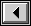# Euler's Method

Euler's method approximates the solution of an initial value problem of the form y' = f(x,y), y(x_0) = y_0.

In the applet below, enter f(x,y), x_0, y_0, and b, where [x_0, b] is the interval over which you want to approximate. Also enter n, the number of subintervals of [x_0, b] you want to use. (Then h = (b - x_0)/n is the step size.) When entering f(x,y), you can use +, -, *, /, ^, (), sin(), cos(), tan(), ln(), log(), asin(), acos(), atan(), pi, e. Take care to remember the * in all multiplications and avoid discontinuities. Then press the "Enter" button. If n > 10, press the "Run" button to get the trajectory traced out by Euler's method. If n < = 10, press the "Step" button repeatedly to get the trajectory traced out by Euler's method one step at a time. Each time there will be a short gray line segment at the end of the incomplete trajectory with slope equal to the derivative of y there, which gives the direction of the next piece of the trajectory.

This applet uses a slightly modified version of the expression parser expr written by Darius Bacon.

source codeBack to the Protas home page